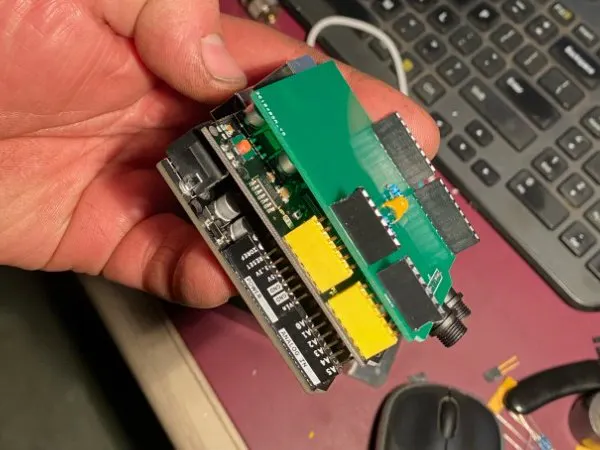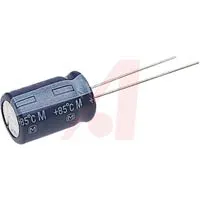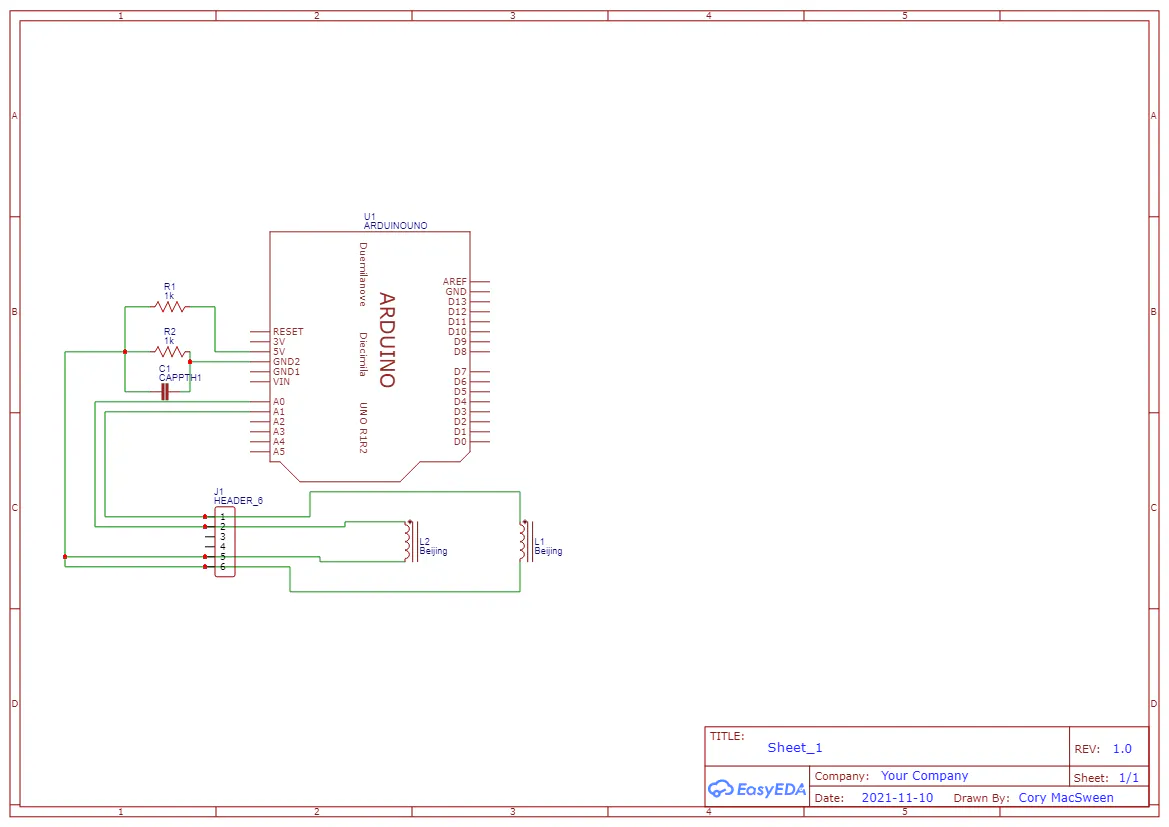Published

# Whole House energy monitor

Whole house energy monitor that monitors a split phase 120/240 service and publishes to blynk.

IntermediateFull instructions provided4,734## Things used in this project

### Hardware componentsArduino UNO
×1
 100 Amp current transformer
×1
 Arduino uno ethernet shield
×1Resistor 10k ohm
×2Capacitor 10 µF
×1
 Project Box
×1
×1
 My custom shield for CT hookup
×1

### Software apps and online servicesBlynk

### Hand tools and fabrication machinesSoldering iron (generic)Drill / Driver, CordlessPlier, Side Cutting

## Schematics

### schematic_uno_energy_monitor_2021-11-21_WrFuMp9doE.png## Code

### Sketch

Arduino
Arduino Energy Monitor Code
```#include <SPI.h>
#include <Ethernet.h>
#include <BlynkSimpleEthernet.h>
#include <TimeLib.h>
#include <WidgetRTC.h>
char auth[] = "your blynk token here"; // Put the token from your blynk project here
double calibration = 0.32; // Modify this to calibrate your sensor o.32 should be accurent for a 100 amp CT
double kilos0;
double kilos1;
double peakkilos;
unsigned long startMillis;
unsigned long endMillis;
double RMSCurrent0;
double RMSCurrent1;
int RMSPower0;
int RMSPower1;
int peakPower0;
int peakPower1;
int TotalRMSPower;
int current0 = 0;
int maxCurrent0 = 0;
int minCurrent0 = 1000;
int current1 = 0;
int maxCurrent1 = 0;
int minCurrent1 = 1000;
int zero0 = 516;
int zero1 = 516;
int Volts = 121.2; // Change this field to the voltage your house is supplied with
BlynkTimer timer;
WidgetRTC rtc;

void setup()
{
Serial.begin(115200);
Blynk.begin(auth, IPAddress(***, ***, ***, ***), 8080); //Replace the astrics with the IP address of your local blynk server
setSyncInterval(10 * 60);
}

void loop()
{
if (Blynk.connected()) {  // If connected run as normal
Blynk.run();
rtc.begin();
timer.run();
} else {
Blynk.connect();
}
displayKilowattHours();
displayCurrent();
displayPower();
if (hour() == 23 && minute() == 59) {
kilos0 = 0;
kilos1 = 0;
}
delay(100);
delay(100);
}

void clockDisplay()
{
String currentTime = String(hour()) + ":" + minute() + ":" + second();
String currentDate = String(day()) + " " + month() + " " + year();
Serial.print("Current time: ");
Serial.println(currentTime);
}

{
maxCurrent0 = zero0;
minCurrent0 = zero0;
maxCurrent1 = zero1;
minCurrent1 = zero1;
for (int i = 0 ; i <= 200 ; i++)
{
if (current0 >= maxCurrent0)
maxCurrent0 = current0;
else if (current0 <= minCurrent0)
minCurrent0 = current0;
if (current1 >= maxCurrent1)
maxCurrent1 = current1;
else if (current1 <= minCurrent1)
minCurrent1 = current1;
}
zero0 = ((maxCurrent0 - minCurrent0) / 2) + minCurrent0;
zero1 = ((maxCurrent1 - minCurrent1) / 2) + minCurrent1;
RMSCurrent0 = (maxCurrent0 - zero0) * calibration;
RMSCurrent1 = (maxCurrent1 - zero1) * calibration;
RMSPower0 = Volts * RMSCurrent0;
RMSPower1 = Volts * RMSCurrent1;
TotalRMSPower = RMSPower0 + RMSPower1;
endMillis = millis();
unsigned long time = (endMillis - startMillis);
kilos0 = kilos0 + (((double)RMSPower0 / 1000) * ((double)time / 3600000));
kilos1 = kilos1 + (((double)RMSPower1 / 1000) * ((double)time / 3600000));
peakkilos = kilos0 + kilos1;
startMillis = millis();
}

void displayKilowattHours()  //Displays all kilowatt hours data
{
Serial.print(kilos0);
Serial.print("kWh          ");
Serial.print(kilos1);
Serial.println("kWh");
Serial.print(peakkilos);
Serial.println("kWh");
Blynk.virtualWrite(V2, peakkilos);
}

void displayCurrent()      //Displays all current data
{
Serial.print(RMSCurrent0);
Serial.print("A          ");
Serial.print(RMSCurrent1);
Serial.println("A");
Blynk.virtualWrite(V0, RMSCurrent0);
Blynk.virtualWrite(V1, RMSCurrent1);
}

void displayPower()      //Displays all watts
{
Serial.print(RMSPower0);
Serial.print("W          ");
Serial.print(RMSPower1);
Serial.print("W          ");
Serial.print(TotalRMSPower);
Serial.println("W");
}
```

## Credits

### Corymacs

0 projects • 1 follower